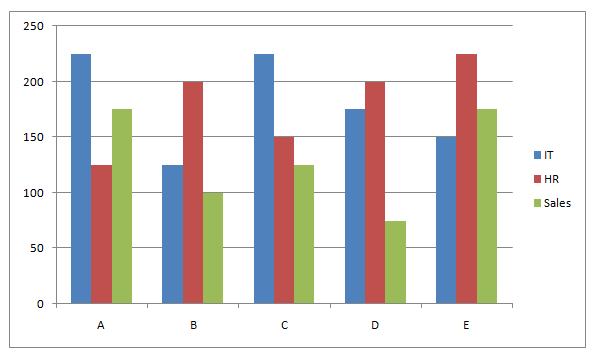# SBI Clerk Prelims 2021 Quantitative Aptitude Questions (Day-37)

Dear Aspirants, Our IBPS Guide team is providing new series of Quantitative Aptitude Questions for SBI Clerk Prelims 2020 so the aspirants can practice it on a daily basis. These questions are framed by our skilled experts after understanding your needs thoroughly. Aspirants can practice these new series questions daily to familiarize with the exact exam pattern and make your preparation effective.

Start Quiz

Approximation

Directions (01-05): What approximate value should come in the place of (?) in the following questions.

1) 42.851 % of 350 + 71.428% of 140 = ? + 49.91

A.200

B.180

C.220

D.250

E.280

2) 8.011 * 35.91 + √360 * √530 = ? + 11.05 * 47.903

A.193

B.197

C.203

D.188

E.183

3) 15.05% of 639.901 + 19.879 % of 420.15 = ? * 8.93

A.15

B.18

C.20

D.24

E.26

4) 145.26 % of 159.971 + 114.97 % of 420.078 = ?+ 414.891

A.250

B.200

C.350

D.300

E.400

5) 32.162% of 549.95 + 1012.02 ÷ 22.87 = ? * 11.091

A.18

B.20

C.22

D.24

E.26

Bar graph

Directions (06-10): Study the following information carefully and answer the questions given below.

The given bar graph shows the number of employees in three different departments in five different companies.6) Total number of employees from A in all the departments together is approximately what percent more than the total number of employees from D in all the departments together?

A.13.34%

B.16.67%

C.12.23%

D.18.89%

E.15.56%

7) What is the ratio of the number of IT employees from B, C and E together to number of HR employees from B, C and E together?

A.19:21

B.20:23

C.21:25

D.22:27

E.17:22

8) Total number sales employees in all the companies together are approximately what percent of the total number of IT employees in all the companies together?

A.72%

B.76%

C.80%

D.84%

E.88%

9) If the ratio of the number of male to female employees in HR department from E is 4:5 and the ratio of the number of male to female employees in sales department from C is 3:2. What is the difference between the number of male and female employees in HR and Sales department together from company C and E?

A.0

B.5

C.2

D.3

E.4

10) The number of IT employees from A is approximately what percent of the number of HR employees from B and C together?

A.60%

B.64%

C.68%

D.72%

E.76%

42.851 % of 350 + 71.428% of 140 = ? + 49.91

150 + 100 = ? + 50

? = 200

8.011 * 35.91 + √360 * √530 = ? + 11.05 * 47.903

288 + 437 = ? + 528

? = 197

15.05% of 639.901 + 19.879 % of 420.15 = ? * 8.93

96 + 84 = ? * 9

? = 20

145.26 % of 159.971 + 114.97 % of 420.078 = ?+ 414.891

232 + 483 = ? + 415

? = 300

32.162% of 549.95 + 1012.02 ÷ 22.87 = ? * 11.091

176 + 44 = ? * 11

? = 20

Required percentage = ((225 + 125 + 175) – (175 + 200 + 75))/(175 + 200 + 75) * 100

= 16.67%

IT employees in B, C and E = 125 + 225 + 150 = 500

HR employees in B, C and E = 200 + 150 + 225 = 575

Required ratio = 500:575

= 20:23

Required percentage = (175 + 100 + 125 + 75 + 175)/(225 + 125 + 225 + 175 + 150) * 100

= 72%

Number of male employees in HR department from E = 225 * 4/9 = 100

Number of female employees in HR department from E = 225 * 5/9 = 125

Number of male employees in Sales department from C = 3/5 * 125 = 75

Number of female employees in Sales department from C = 2/5 * 125 = 50

Required Difference = (125 + 50) – (100 + 75) = 0